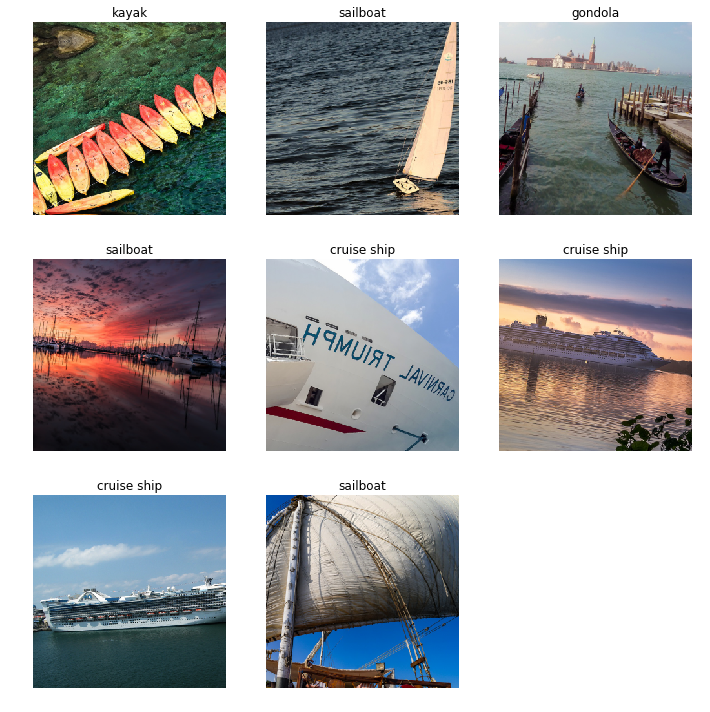#### Load the drive helper and mount

In [ ]:
``````from google.colab import drive

# this will prompt for authorization
drive.mount('/content/gdrive',force_remount=True)

# dataset file path
root_path = "gdrive/My Drive/colab_folder/"``````

#### Upload the Kaggle API file to Google Colab

In [ ]:
``````# upload the kaggle API key file
from google.colab import files
files.upload()
``````
In [ ]:
``````# move the API key to the kaggle folder.Give permissions to change.
!mkdir -p ~/.kaggle/ && mv kaggle.json ~/.kaggle/ && chmod 600 ~/.kaggle/kaggle.json``````

#### Download the dataset from Kaggle and unzip

In [ ]:
``````# search datasets by name
!kaggle datasets list -s boat``````
In [ ]:
``````# download the dataset
!kaggle datasets download -d clorichel/boat-types-recognition -p /content/gdrive/My\ Drive/colab_folder/datasets
``````
In [ ]:
``````# print the current working directory
!pwd
``````
```/content ```
In [ ]:
``````# change the directory to the folder holding the dataset
import os
os.chdir('gdrive/My Drive/colab_folder/datasets')``````
In [ ]:
``````# get a list of files and directories
!ls
``````
In [ ]:
``````# create a new directory
!mkdir boats
``````
In [ ]:
``````# unzip files to the new directory
!unzip -q boat-types-recognition.zip ``````
In [ ]:
``````# check the list
!ls
``````

#### fastai image classification

In [ ]:
``````# directives
%reload_ext autoreload
%autoreload 2
%matplotlib inline
``````
In [ ]:
``````# import packages
from fastai import *
from fastai.vision import *
from fastai.metrics import accuracy``````
In [ ]:
``bs=8 # batch_size greater than this caused memory issues``
In [ ]:
``````# prepare and load the training and validation datasets
np.random.seed(12)
data= ImageDataBunch.from_folder(Path('boats/'), train='.',valid_pct=0.20,size=299, bs=bs, ds_tfms=get_transforms()).normalize(imagenet_stats)``````
In [ ]:
``data``
Out:
``````ImageDataBunch;

Train: LabelList (1170 items)
x: ImageList
Image (3, 299, 299),Image (3, 299, 299),Image (3, 299, 299),Image (3, 299, 299),Image (3, 299, 299)
y: CategoryList
cruise ship,cruise ship,cruise ship,cruise ship,cruise ship
Path: boats;

Valid: LabelList (292 items)
x: ImageList
Image (3, 299, 299),Image (3, 299, 299),Image (3, 299, 299),Image (3, 299, 299),Image (3, 299, 299)
y: CategoryList
ferry boat,paper boat,sailboat,sailboat,freight boat
Path: boats;

Test: None``````
In [ ]:
``````# visualize a few examples
data.show_batch(rows=3,figsize=(10,10))``````In [ ]:
``````# look at the labels
data.classes
``````
Out:
``````['buoy',
'cruise ship',
'ferry boat',
'freight boat',
'gondola',
'inflatable boat',
'kayak',
'paper boat',
'sailboat']``````
In [ ]:
``````# look at the number of classes, training and validation datasets
data.c, len(data.train_ds), len(data.valid_ds) ``````
Out:
``(9, 1170, 292)``
In [ ]:
``````# model and metrics definition
learn=cnn_learner(data,models.resnet152,metrics=accuracy)
``````
```Downloading: "https://download.pytorch.org/models/resnet152-b121ed2d.pth" to /root/.cache/torch/checkpoints/resnet152-b121ed2d.pth 100%|██████████| 241530880/241530880 [00:16<00:00, 14901974.81it/s] ```
In [ ]:
``learn.model``
In [ ]:
``````# train for 4 cycles through the data
learn.fit_one_cycle(4) ``````
In [ ]:
``````# save the model
learn.save('stage-1')``````
In [ ]:
``````# plot the top losses
interp=ClassificationInterpretation.from_learner(learn)
interp.plot_top_losses(9, figsize=(10,10))``````